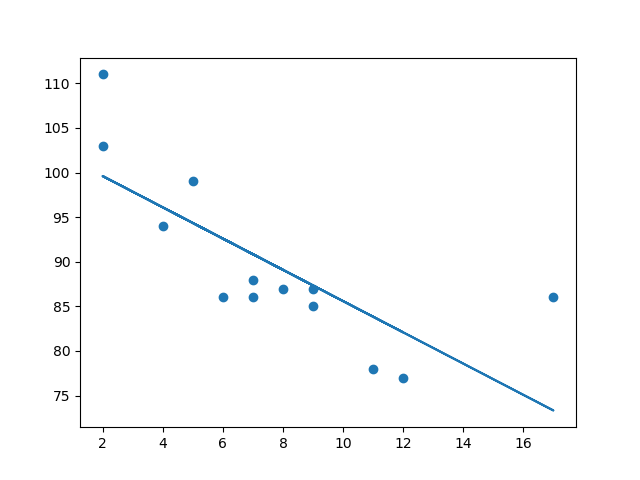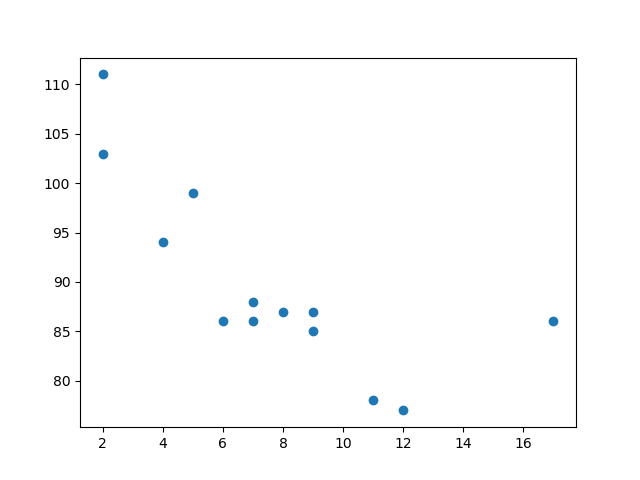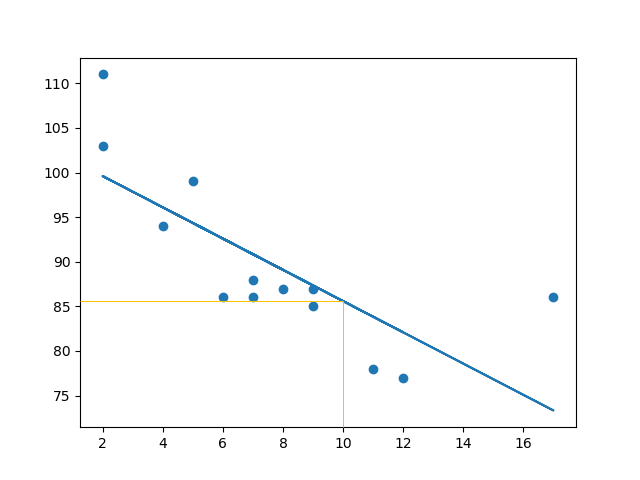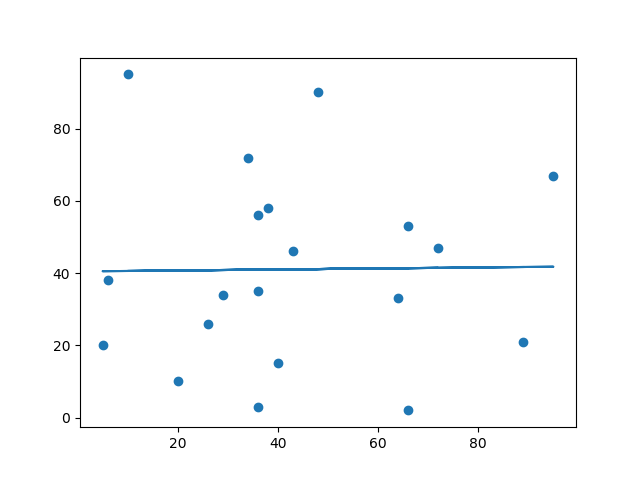## Python Tutorial

Python HOME Python Intro Python Get Started Python Syntax Python Comments Python Variables Python Data Types Python Numbers Python Casting Python Strings Python Booleans Python Operators Python Lists Python Tuples Python Sets Python Dictionaries Python If...Else Python While Loops Python For Loops Python Functions Python Lambda Python Arrays Python Classes/Objects Python Inheritance Python Iterators Python Polymorphism Python Scope Python Modules Python Dates Python Math Python JSON Python RegEx Python PIP Python Try...Except Python User Input Python String Formatting

## File Handling

Python File Handling Python Read Files Python Write/Create Files Python Delete Files

## Python Modules

NumPy Tutorial Pandas Tutorial SciPy Tutorial Django Tutorial

## Python Matplotlib

Matplotlib Intro Matplotlib Get Started Matplotlib Pyplot Matplotlib Plotting Matplotlib Markers Matplotlib Line Matplotlib Labels Matplotlib Grid Matplotlib Subplot Matplotlib Scatter Matplotlib Bars Matplotlib Histograms Matplotlib Pie Charts

## Machine Learning

Getting Started Mean Median Mode Standard Deviation Percentile Data Distribution Normal Data Distribution Scatter Plot Linear Regression Polynomial Regression Multiple Regression Scale Train/Test Decision Tree Confusion Matrix Hierarchical Clustering Logistic Regression Grid Search Categorical Data K-means Bootstrap Aggregation Cross Validation AUC - ROC Curve K-nearest neighbors

## Python MySQL

MySQL Get Started MySQL Create Database MySQL Create Table MySQL Insert MySQL Select MySQL Where MySQL Order By MySQL Delete MySQL Drop Table MySQL Update MySQL Limit MySQL Join

## Python MongoDB

MongoDB Get Started MongoDB Create Database MongoDB Create Collection MongoDB Insert MongoDB Find MongoDB Query MongoDB Sort MongoDB Delete MongoDB Drop Collection MongoDB Update MongoDB Limit

## Python Reference

Python Overview Python Built-in Functions Python String Methods Python List Methods Python Dictionary Methods Python Tuple Methods Python Set Methods Python File Methods Python Keywords Python Exceptions Python Glossary

## Module Reference

Random Module Requests Module Statistics Module Math Module cMath Module

## Python How To

Remove List Duplicates Reverse a String Add Two Numbers

## Python Examples

Python Examples Python Compiler Python Exercises Python Quiz Python Bootcamp Python Certificate

# Machine Learning - Linear Regression

## Regression

The term regression is used when you try to find the relationship between variables.

In Machine Learning, and in statistical modeling, that relationship is used to predict the outcome of future events.

## Linear Regression

Linear regression uses the relationship between the data-points to draw a straight line through all them.

This line can be used to predict future values.In Machine Learning, predicting the future is very important.

## How Does it Work?

Python has methods for finding a relationship between data-points and to draw a line of linear regression. We will show you how to use these methods instead of going through the mathematic formula.

In the example below, the x-axis represents age, and the y-axis represents speed. We have registered the age and speed of 13 cars as they were passing a tollbooth. Let us see if the data we collected could be used in a linear regression:

### Example

Start by drawing a scatter plot:

import matplotlib.pyplot as plt

x = [5,7,8,7,2,17,2,9,4,11,12,9,6]
y = [99,86,87,88,111,86,103,87,94,78,77,85,86]

plt.scatter(x, y)
plt.show()

### Result:Run example »

### Example

Import `scipy` and draw the line of Linear Regression:

import matplotlib.pyplot as plt
from scipy import stats

x = [5,7,8,7,2,17,2,9,4,11,12,9,6]
y = [99,86,87,88,111,86,103,87,94,78,77,85,86]

slope, intercept, r, p, std_err = stats.linregress(x, y)

def myfunc(x):
return slope * x + intercept

mymodel = list(map(myfunc, x))

plt.scatter(x, y)
plt.plot(x, mymodel)
plt.show()

### Result:Run example »

### Example Explained

Import the modules you need.

You can learn about the Matplotlib module in our Matplotlib Tutorial.

You can learn about the SciPy module in our SciPy Tutorial.

```import matplotlib.pyplot as pltfrom scipy import stats```

Create the arrays that represent the values of the x and y axis:

``` x = [5,7,8,7,2,17,2,9,4,11,12,9,6]y = [99,86,87,88,111,86,103,87,94,78,77,85,86] ```

Execute a method that returns some important key values of Linear Regression:

``` slope, intercept, r, p, std_err = stats.linregress(x, y)```

Create a function that uses the `slope` and `intercept` values to return a new value. This new value represents where on the y-axis the corresponding x value will be placed:

``` def myfunc(x):   return slope * x + intercept```

Run each value of the x array through the function. This will result in a new array with new values for the y-axis:

``` mymodel = list(map(myfunc, x))```

Draw the original scatter plot:

``` plt.scatter(x, y)```

Draw the line of linear regression:

``` plt.plot(x, mymodel)```

Display the diagram:

``` plt.show()```

## R for Relationship

It is important to know how the relationship between the values of the x-axis and the values of the y-axis is, if there are no relationship the linear regression can not be used to predict anything.

This relationship - the coefficient of correlation - is called `r`.

The `r` value ranges from -1 to 1, where 0 means no relationship, and 1 (and -1) means 100% related.

Python and the Scipy module will compute this value for you, all you have to do is feed it with the x and y values.

### Example

How well does my data fit in a linear regression?

from scipy import stats

x = [5,7,8,7,2,17,2,9,4,11,12,9,6]
y = [99,86,87,88,111,86,103,87,94,78,77,85,86]

slope, intercept, r, p, std_err = stats.linregress(x, y)

print(r)
Try it Yourself »

Note: The result -0.76 shows that there is a relationship, not perfect, but it indicates that we could use linear regression in future predictions.

## Predict Future Values

Now we can use the information we have gathered to predict future values.

Example: Let us try to predict the speed of a 10 years old car.

To do so, we need the same `myfunc()` function from the example above:

``` def myfunc(x):   return slope * x + intercept```

### Example

Predict the speed of a 10 years old car:

from scipy import stats

x = [5,7,8,7,2,17,2,9,4,11,12,9,6]
y = [99,86,87,88,111,86,103,87,94,78,77,85,86]

slope, intercept, r, p, std_err = stats.linregress(x, y)

def myfunc(x):
return slope * x + intercept

speed = myfunc(10)

print(speed)
Run example »

The example predicted a speed at 85.6, which we also could read from the diagram:Let us create an example where linear regression would not be the best method to predict future values.

### Example

These values for the x- and y-axis should result in a very bad fit for linear regression:

import matplotlib.pyplot as plt
from scipy import stats

x = [89,43,36,36,95,10,66,34,38,20,26,29,48,64,6,5,36,66,72,40]
y = [21,46,3,35,67,95,53,72,58,10,26,34,90,33,38,20,56,2,47,15]

slope, intercept, r, p, std_err = stats.linregress(x, y)

def myfunc(x):
return slope * x + intercept

mymodel = list(map(myfunc, x))

plt.scatter(x, y)
plt.plot(x, mymodel)
plt.show()

### Result:Run example »

And the `r` for relationship?

### Example

You should get a very low `r` value.

import numpy
from scipy import stats

x = [89,43,36,36,95,10,66,34,38,20,26,29,48,64,6,5,36,66,72,40]
y = [21,46,3,35,67,95,53,72,58,10,26,34,90,33,38,20,56,2,47,15]

slope, intercept, r, p, std_err = stats.linregress(x, y)

print(r)
Try it Yourself »

The result: 0.013 indicates a very bad relationship, and tells us that this data set is not suitable for linear regression.

W3Schools is optimized for learning and training. Examples might be simplified to improve reading and learning. Tutorials, references, and examples are constantly reviewed to avoid errors, but we cannot warrant full correctness of all content. While using W3Schools, you agree to have read and accepted our terms of use, cookie and privacy policy.